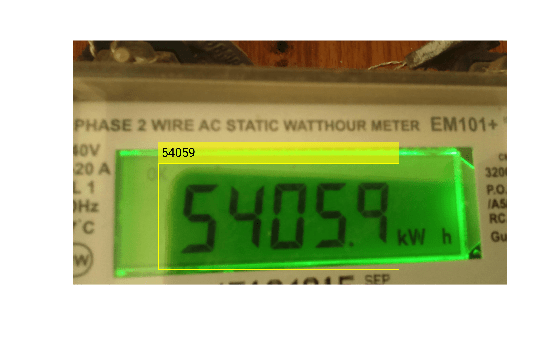# quantizeOCR

Quantize OCR model

Since R2023a

## Syntax

``outputFileName = quantizeOCR(inputFileName,outputModelName)``
``outputFileName = quantizeOCR(___,OutputLocation=location)``

## Description

example

````outputFileName = quantizeOCR(inputFileName,outputModelName)` quantizes the specified optical character recognition (OCR) model `inputFileName` and stores it in the present working directory, specified by `outputModelName`.`quantizeOCR` quantizes the weights of an OCR model to produce a new OCR model that is faster and use less memory, but at the cost of reduced accuracy in results.```
````outputFileName = quantizeOCR(___,OutputLocation=location)` specifies the location to store the returned quantized model, in addition to the input arguments from the previous syntax.. By default, `location` is set to the present working directory. Specify `location` as a character vector or string scalar of the absolute or relative path for the desired output location```

## Examples

collapse all

This example shows how to quantize the seven-segment OCR model that ships with the Computer Vision Toolbox™.

Specify the fullpath to the OCR model.

```model = fullfile(toolboxdir("vision"),"visionutilities",... "tessdata_best","seven_segment.traineddata");```

Specify a filename to save the quantized OCR model.

`outputModelName = "quantizedModel";`

Quantize the OCR model.

`outputModel = quantizeOCR(model,outputModelName);`

Use the quantized model to perform OCR on a test image and visualize the results.

```I = imread("sevSegDisp.jpg"); roi = [506 725 1418 626]; ocrResults = ocr(I,roi,Model=outputModel,LayoutAnalysis="block"); Iocr = insertObjectAnnotation(I,"rectangle",... ocrResults.WordBoundingBoxes,ocrResults.Words,... LineWidth=5,FontSize=72); imshow(Iocr)```This example shows how to improve runtime performance of an OCR model with quantization. This can be useful when deploying an OCR model in resource constrained systems.

Specify the fullpath to the OCR model.

```originalModel = fullfile(toolboxdir("vision"),"visionutilities",... "tessdata_best","seven_segment.traineddata");```

Specify a filename to save the quantized OCR model.

`outputModelName = "quantizedModel";`

Quantize the OCR model.

`quantizedModel = quantizeOCR(originalModel,outputModelName);`

Compare the runtime performance of the quantized model against the original model.

```I = imread("sevSegDisp.jpg"); roi = [506 725 1418 626]; fOCR = @() ocr(I, roi, Model=originalModel, LayoutAnalysis="block"); tOCR = timeit(fOCR); fQuantizedOCR = @() ocr(I, roi, Model=quantizedModel, LayoutAnalysis="block"); tQuantizedOCR = timeit(fQuantizedOCR); perfRatio = tOCR/tQuantizedOCR; disp("Quantized model is " + perfRatio + "x faster");```
```Quantized model is 2.3637x faster ```

Compare file size of the quantized model with that of the original model.

```originalModelFile = dir(originalModel); originalModelFileSizeInMB = originalModelFile.bytes/1000000; quantizedModelFile = dir(quantizedModel); quantizedModelFileSizeInMB = quantizedModelFile.bytes/1000000; sizeRatio = originalModelFileSizeInMB/quantizedModelFileSizeInMB; disp("Quantized model is " + sizeRatio + "x smaller");```
```Quantized model is 7.8483x smaller ```

While the quantized model is smaller and faster than the original model, these advantages of quantization comes at the expense of accuracy. To understand this trade-off, compare the accuracy of the two models by evaluating them against the YUVA EB dataset.

```datasetURL = "https://ssd.mathworks.com/supportfiles/vision/data/7SegmentImages.zip"; datasetZip = "7SegmentImages.zip"; if ~exist(datasetZip,"file") disp("Downloading evaluation data set (" + datasetZip + " - 96 MB)..."); websave(datasetZip,datasetURL); end```
```Downloading evaluation data set (7SegmentImages.zip - 96 MB)... ```
`datasetFiles = unzip(datasetZip);`

Load ground truth to be used for evaluation.

```ld = load("7SegmentGtruth.mat"); gTruth = ld.gTruth;```

Create datastores that contain images, bounding boxes and text labels from the `groundTruth` object using the `ocrTrainingData` function with the label and attribute names used during labeling.

```labelName = "Text"; attributeName = "Digits"; [imds,boxds,txtds] = ocrTrainingData(gTruth,labelName,attributeName); cds = combine(imds,boxds,txtds);```

Run the two models on the dataset and evaluate recognition accuracy using `evaluateOCR`.

```originalResult = ocr(cds, Model=originalModel); quantizedResult = ocr(cds, Model=quantizedModel); metricsOriginal = evaluateOCR(originalResult, cds);```
```Evaluating ocr results ---------------------- * Selected metrics: character error rate, word error rate. * Processed 119 images. * Finalizing... Done. * Data set metrics: CharacterErrorRate WordErrorRate __________________ _____________ 0.082195 0.19958 ```
`metricsQuantized = evaluateOCR(quantizedResult, cds);`
```Evaluating ocr results ---------------------- * Selected metrics: character error rate, word error rate. * Processed 119 images. * Finalizing... Done. * Data set metrics: CharacterErrorRate WordErrorRate __________________ _____________ 0.13018 0.31933 ```

Display the model accuracies.

```originalModelAccuracy = 100*(1-metricsOriginal.DataSetMetrics.CharacterErrorRate); quantizedModelAccuracy = 100*(1-metricsQuantized.DataSetMetrics.CharacterErrorRate); disp("Original model accuracy = " + originalModelAccuracy + "%")```
```Original model accuracy = 91.7805% ```
`disp("Quantized model accuracy = " + quantizedModelAccuracy + "%")`
```Quantized model accuracy = 86.9816% ```

Tabulate the quantitative results.

```trainedModelResults = [originalModelAccuracy; originalModelFileSizeInMB; tOCR]; quantizedModelResults = [quantizedModelAccuracy; quantizedModelFileSizeInMB; tQuantizedOCR]; table(trainedModelResults, quantizedModelResults, ... VariableNames=["OriginalModel", "QuantizedModel"], ... RowNames=["Accuracy (in %)", "File Size (in MB)", "Runtime (in seconds)"])```
```ans=3×2 table OriginalModel QuantizedModel _____________ ______________ Accuracy (in %) 91.781 86.982 File Size (in MB) 11.297 1.4394 Runtime (in seconds) 0.12307 0.052069 ```

## Input Arguments

collapse all

Name of the OCR model file, specified as a string scalar or a character vector. If `inputFileName` does not include the full path, the function searches for the file in the current folder and along the MATLAB® path. If you do not specify a file extension, the function appends `.traineddata`.

Output quantized model name, specified as a string scalar or character vector.

## Output Arguments

collapse all

Name of quantized OCR model file, specified as a string scalar or character vector.

## Version History

Introduced in R2023a